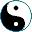State Machine (continued)
<<
>>
 Yes, how about mX : (A × (A × X)A)A → (A × X)A? We have another collection of state machines, mX : (A × (A × X)A)A, which has A as a state set, and which outputs to another collection of state machines (this one too has A as a state set, and X as output set).How do we find for such a compound state machine a match in a state machine on A? Write it in Lisp: ```(define (mx f) (let (tr1 out1) ((car f)(cadr f)));two components of f:A → (A × (A × X)A (lambda (a) (let a1 (tr1 a));state after first transition (let f2 (out1 a)) ;mapped a state to another state machine (let (tr2 out2) ((car f2) (cadr f2))) ;second machine components (list (tr2 a1) (out2 a1))))``` See what happens here: we have a function from A to A×(A×X)A, which consists of transition A → A and output A → (A×X)A, that is, for each a we have another state a1 and an output function; the resulting function from A to A×X should just apply that output function to the new state. Boring Exercise: Prove that this is actually a monad.